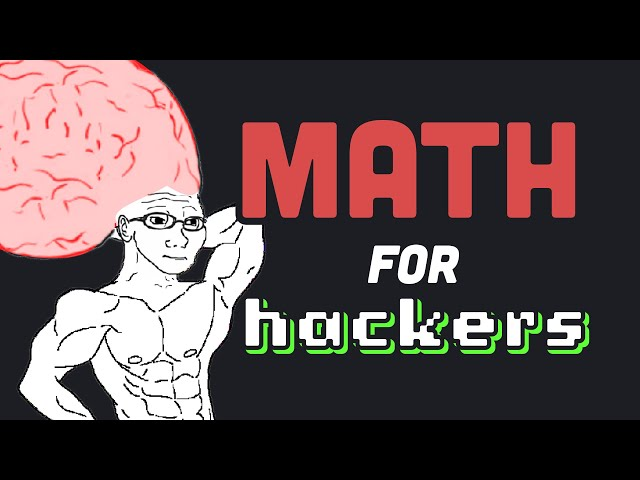# Mastering Math for Efficient Programming: 10 Essential ConceptsTLDR Learning math as a programmer is essential for unlocking the secrets of the physical universe, and understanding concepts such as Boolean algebra, binary numeral systems, rounding errors, combinatorics, graphs, and linear algebra is crucial for building efficient algorithms and deep neural networks.

## Key insights

• 🔮
Learning math can unlock the secrets of the entire physical Universe, making complicated magic like computer graphics and neural networks easier to understand.
• 💻
Boolean algebra is a fundamental concept in programming, used almost every time you write code.
• 💻
Computers work off of a base 2 numeral system, which is why understanding other bases like hexadecimal is important for programmers.
• 🔢
Understanding logarithms and exponentiation is crucial for many algorithms, but the choice of base can vary depending on the situation.
• 🤯
Understanding set theory is crucial for programmers working with relational databases and can lead to complex joins and unions.
• 💻
Understanding complexity theory is essential for technical interviews and is just a good thing to think about in general when writing algorithms.
• 🧮
Understanding the difference between linear and logistic regression is crucial for programmers working on prediction and classification problems.
• 🤯
The underlying math behind graphics and computer magic is surprisingly simple, but requires a deep understanding of linear transformations and matrix multiplication.

## Q&A

• Why is learning math important for programmers?

Learning math as a programmer can make complicated technologies easier to understand and unlock the secrets of the physical universe.

• What is Boolean algebra?

Boolean algebra is used in coding and involves binary variables with true or false values, and three operators (and, or, not) to represent logic in code.

• What numeral system do computers use?

Computers use base 2 (binary) numeral system, but other bases like hexadecimal and base64 are used to represent binary values in a more concise way.

• What is combinatorics?

Combinatorics is a branch of discrete math that deals with counting and understanding patterns in combinations and permutations, used in various fields such as app algorithms and database partitioning.

• Why is linear algebra important for programmers?

Linear algebra is important for computer graphics and deep neural networks, as it is used to perform transformations, but it requires a lot of computing power.

## Timestamped Summary

• 🧮
00:00
Learning math as a programmer unlocks the secrets of the physical universe.
• 💻
00:41
Boolean algebra uses binary variables and logic operators to represent code, visualized with Venn diagrams and truth tables.
• 💻
01:27
Computers use base 2 numeral system to represent binary values, while humans use base 10, and other bases like hexadecimal and base64 are used for more concise representation.
• 💻
02:39
Rounding errors can occur in computer science when using floating point numbers and logarithmic functions, but knowing the number of times a log is cut can help calculate its original length.
• 🔢
04:17
Combinatorics is the math of counting and patterns, used in app algorithms, database partitioning, and nature.
• 📊
05:42
Understanding graphs is important for building efficient algorithms, especially in AI.
• 📈
07:16
Linear regression predicts continuous values, 📊 logistic regression is used for classification, and understanding linear algebra is important for computer graphics and deep neural networks.
• 💻
08:32
Linear algebra is crucial in graphics, cryptography, and deep neural networks, but demands significant computing power.Report the articleThank you for the feedback